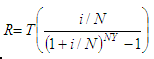# Calculate Future Value - Regular Deposits

 In this article we’re going to develop a script to see how to calculate the future value that regular deposits may produce. This code calculates the amount required as a regular deposit to provide a stated future value in a specified time period. All deposits are equal. It’s necessary for you to supply the future value, the nominal interest rate, the number of deposits per year and the number of years.

The calculation for regular deposits is based on the following formula:where:
R = amount of regular deposit
T = future value
i = nominal interest rate
N = number of deposits per year
Y = number of years

This is our simple Matlab function or script to calculate the above formula:

function r = calc_future_value(t, it, n, y)
it = it/(n*100);
r = t*it/((1+it)^(n*y)-1);

We can create another script to test and run the above m-file:

clc; clear; format bank

t = input('Enter total value after Y years: ');
it = input(
'Enter nominal interest rate: ');
n = input(
'Enter number of deposits per year: ');
y = input(
'Enter number of years: ');

regular_deposits = calc_future_value(t, it, n, y)

Example:

Jackie would like to have \$1000 at the end of one year in a savings account. How much must she deposit each month at 8% interest to achieve it?

We run our test code and get:

Enter total value after Y years: 1000
Enter nominal interest rate: 8
Enter number of deposits per year: 12
Enter number of years: 1

The result is:

regular_deposits = 80.32

From 'Calculate Future Value' to home

From 'Calculate Future Value' to 'Finance Formulas'